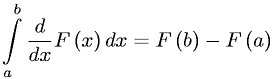Equations > Calculus > Integration/Integrals > Fundamental Theorem of Integrals of Derivatives

### Fundamental Theorem of Integrals of DerivativesLatex Code:

MathML Code:

 $\underset{a}{\overset{b}{\int }}\fracd\mathrm{dx}F\left(x\right)\mathrm{dx}=F\left(b\right)-F\left(a\right)$

MathType 5.0: